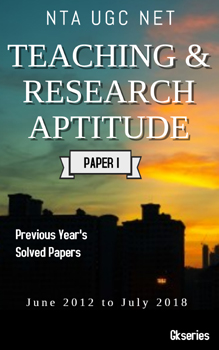# Discrete Mathematics - Questions & Answers for Competitive Exams | GkSeries

Questions
1. If P then Q is called _________ statement
• [A] Conjunction
• [B] disjunction
• [C] conditional
• [D] bi conditional

2. A relation R in a set X is symmetric if _______
• [A] xRy, yRz => xRz.
• [B] xRy
• [C] xRy=>yRx
• [D] xRx

3. If R is reflexive, symmetric and transitive then the relation is said to be ________.
• [A] Binary relation
• [B] Compatibility relation
• [C] Equivalence relation
• [D] Partial order relation

4. If there are more than 2 LMD for a string then it is said to be ___________.
• [A] Ambigious
• [B] unambigious
• [C] language
• [D] finite state automata

5. The specification of proper construction of a sentence is called ________.
• [A] alphabet
• [B] letter
• [C] syntax
• [D] word

6. Context free grammar is also known as _______ grammar.
• [A] type 0
• [B] type 1
• [C] type 2
• [D] type 3

7. Accepting states are denoted by ________.
• [A] circle
• [B] an arrow mark
• [C] double circle
• [D] straight line

8. The set of all finite words over E is denoted by ________.
• [A] E+
• [B] E*
• [C] E
• [D]E-

9. The composition of function is associative but not _________.
• [A] commutative
• [B] associative
• [C] distributive
• [D] idempotent

10. A sum of the variables and their negations in a formula is called ________
• [A] elementary sum
• [B] elementary product
• [C] cnf
• [D] dnf#### NTA UGC NET HISTORY E-BOOK – PREVIOUS YEAR’S SOLVED PAPERS(JUNE 2012 TO JULY 2018)#### NTA UGC NET ADULT EDUCATION E-BOOK – PREVIOUS YEAR’S SOLVED PAPERS(JUNE 2012 TO JULY 2018)#### NTA UGC NET ANTHROPOLOGY E-BOOK – PREVIOUS YEAR’S SOLVED PAPERS(JUNE 2012 TO JULY 2018)#### NTA UGC NET TEACHING & RESEARCH APTITUDE E-BOOK – PREVIOUS YEAR’S SOLVED PAPERS(JUNE 2012 TO JULY 2018)

11. A graph in which every vertex has same degree is called _________ graph.
• [A] regular
• [B] simple
• [C] complete
• [D] null

12. The number of vertices of odd degree in a graph is always ________.
• [A] odd
• [B] even
• [C] zero
• [D] one